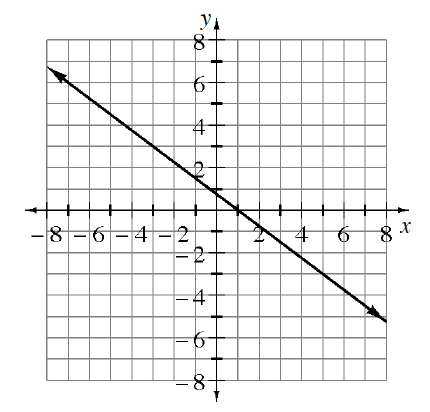Home > ACC7 > Chapter cc37 > Lesson cc37.2.4 > Problem7-73

7-73.Ella and her study team are arguing about the slope of the line in the graph below. They have come up with four different answers: $\frac { 3 } { 4 }$, $- \frac { 4 } { 3 }$, $- \frac { 3 } { 4 }$ and $\frac { 4 } { 3 }$. Which slope is correct? Justify your answer. Homework Help ✎Remember that the slope can be written as $\frac{\text{change in}\ y}{\text{change in}\ x}$

On this line as the $x$-value increases, does the $y$-value decrease or increase?
Decreasing makes the slope negative.
Increasing makes the slope positive.

Try answering this problem by going from the point (−3, 3) to the point (1, 0).